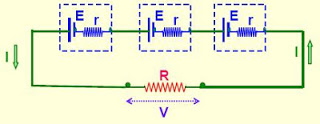### Combination of cells in series

When external resistance is negligible in comparison to the internal resistance, then the cells are connected in parallel to get maximum current.Cells are connected in series when they are joined end to end so that the same quantity of electricity must flow through each cell.
1. The emf of the battery is the sum of the individual emfs
2. The current in each cell is the same and is identical with the current in the entire arrangement.
3. The total internal resistance of the battery is the sum of the individual internal resistances.
Total emf of the battery = nE (for n no. of identical cells)
Total Internal resistance of the battery = nr
Total resistance of the circuit = nr + R

(i) If R << nr, then I = E / r (ii) If nr << R, then I = n (E / R)

When internal resistance is negligible in comparison to the external resistance, then the cells are
connected in series to get maximum current.

### Combination of cells in Parallel

Cells are said to be connected in parallel when they are joined positive to positive and negative to negative such that current is divided between the cells.1. The emf of the battery is the same as that of a single cell.
2. The current in the external circuit is divided equally among the cells.
3. The reciprocal of the total internal resistance is the sum of the reciprocals of the individual internal resistances.
Total emf of the battery = E
Total Internal resistance of the battery = r / n
Total resistance of the circuit = (r / n) + R

(i) If R << r/n, then I = n(E / r) (ii) If r/n << R, then I = E / R

How useful was this post?

Click on a star to rate it!

Average rating 3.2 / 5. Vote count: 100

No votes so far! Be the first to rate this post.

As you found this post useful...

Follow us on social media!

We are sorry that this post was not useful for you!

Let us improve this post!

Tell us how we can improve this post? Please mention your Email so that we can contact you for better feedback.